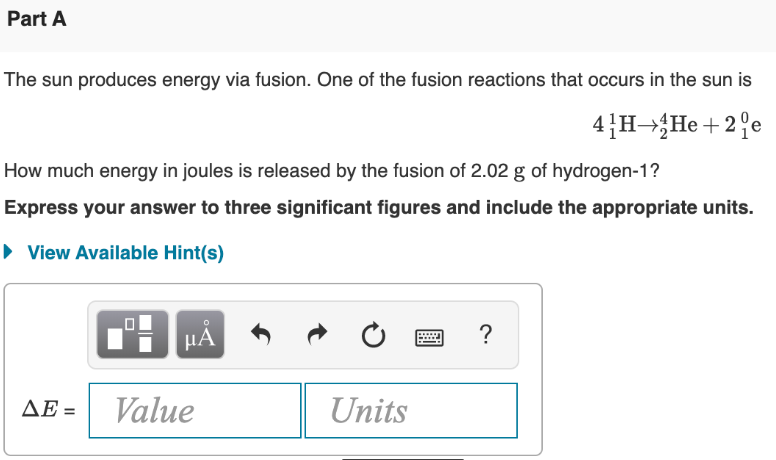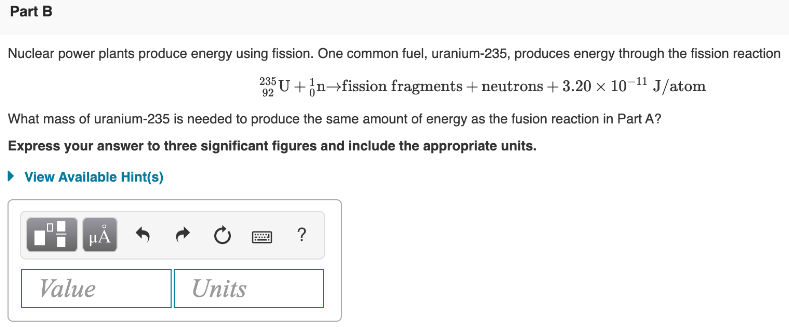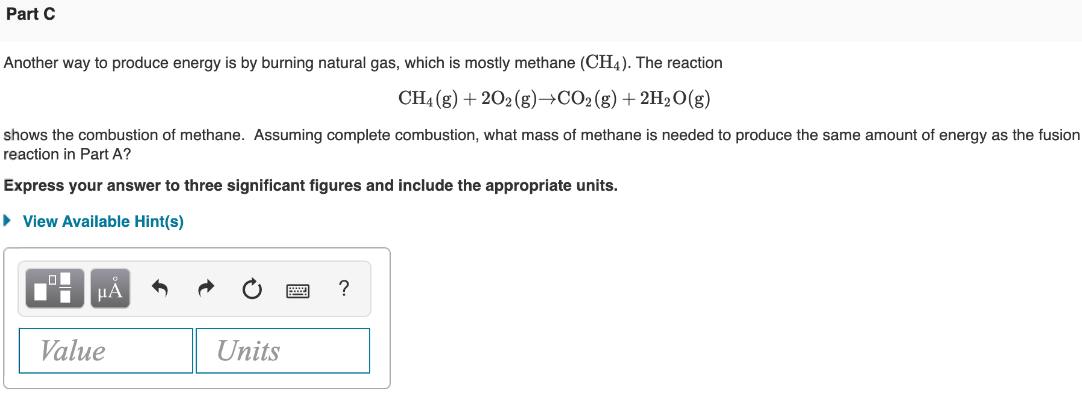# The sun produces energy via fusion. One of the fusion reactions that occurs in the sun is 4 1-H → 4-He + 2 0-e How much energy in joules is released by the fusion of 2.02 g of hydrogen-1? Express your answer to three significant figures and include the appropriate units. Nuclear power plants produce energy using fission. One common fuel, uranium-235, produces energy through the fission reaction 235-U + 1-n→fission fragments + neutrons + 3.20 x 10^-11 J/atom What mass of uranium-235 is needed to produce the same amount of energy as the fusion reaction in Part A? Express your answer to three significant figures and include the appropriate units. Another way to produce energy is by burning natural gas, which is mostly methane (CH4). The reaction CH4(g) + 2O2(g)→CO2(g) + 2H2O(g) shows the combustion of methane. Assuming complete combustion, what mass of methane is needed to produce the same amount of energy as the fusion reaction in Part A? Express your answer to three significant figures and include the appropriate units.